# Difference between Lorentz and Magnetkraft

## Lorentz force explanation

We will deal with the Lorentz force in this article. We will explain to you what is meant by the Lorentz force and first of all provide a number of basics. This article belongs to the field of physics or electrical engineering.The Lorentz force is the force that acts on individual moving charge carriers in a magnetic field. But what does that actually mean? To understand exactly this, let's look at some basics in this area. So let's first take a look at a magnet and a magnetic field. Because that is exactly the basis for understanding the explanation for the Lorentz force.

Show:

### Understanding Lorentz Force Basics

Everyone has probably held a magnet in their hand at some point. With this you can do a piece iron attract. The magnet thus exerts a force on the iron so that it moves. But what is the definition of a magnet? The answer: magnets are bodies that magnetically influence other bodies in their environment. Or to put it another way: magnets (only) exert forces on ferromagnetic substances (e.g. iron, cobalt or nickel).

Every magnet has something called Pole, a north pole and a south pole. These names come from the compass needles, which point with their ends to the magnetic poles of the earth. The same poles repel each other, different ones attract each other. Bar magnets and horseshoe magnets also have a north pole and a south pole. The following graphic shows a horseshoe magnet.

Example horseshoe magnet: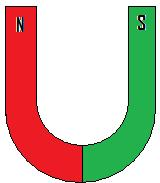Magnetic effects can only be determined in the area of ​​the magnetic field. With field lines one can graphically represent the direction of the magnetic force for each point of such a magnetic field. These field lines have the following properties:

• The magnetic field lines run from the north pole to the south pole
• The direction of the field lines corresponds to the direction in which a sample north pole would move.
• Magnetic field lines do not intersect
• A higher density of the field lines means that there is a stronger magnetic field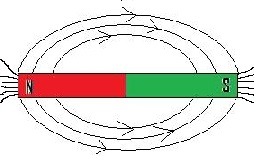Show:

### Lorentz force definition and explanation

The definition for the Lorentz force is: The Lorentz force is the force that acts on individual moving charge carriers in a magnetic field. And let's take a closer look at this with the help of some graphics. Explanation: We have a magnet with a north and south pole, in which the field lines are entered. It will look like that: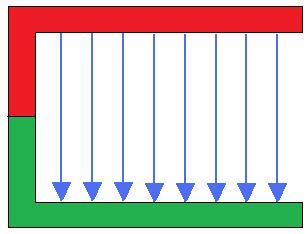We are now holding a conductor (shown in black) between the north and south poles. The electricity is first out: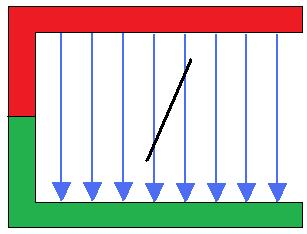And now we activate the current (note + and -). This creates a Lorentz force that moves the conductor to the side. The force acts to the right, so the ladder goes to the right.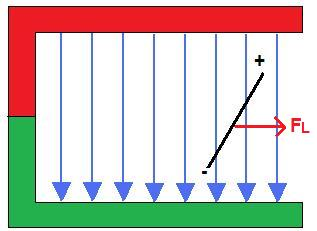And now the polarity is reversed (so we swap + and -). As a result, the Lorentz force acts to the left, and the ladder thus goes to the left.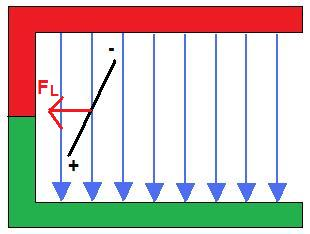### Lorentz force formula

Some formulas are still missing. The first formula can be used to calculate the Lorentz force, with the other corresponding variables for the formula of the Lorentz force. Condition: Assuming that the motion of charged particles is perpendicular to the direction of the magnetic field, the magnitude of the Lorentz force can be calculated using the following formula.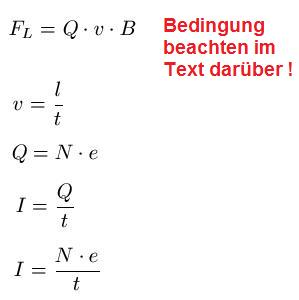Where:

• "F.L."the Lorentz force in Newtons
• "Q" is the total charge on the particles in coulombs
• "v" is the speed of the charged particles in meters per second
• "B" is the magnetic flux density in Newtons per ammeter
• "l" is the length of the conductor in meters
• "t" is the time in seconds
• "N" is the number of charged particles, without units
• "e" is the charge on a particle in coulombs
• "I is the current in amperes

If the charged particles do not move perpendicularly, but at an angle α (spoken: alpha) to the direction of the magnetic field, then the formula changes to: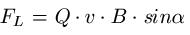### Left hand rule and right hand rule

The right-hand rule and the left-hand rule are rules of thumb for the direction of the magnetic field that is generated by a current-carrying conductor. These rules can be used to determine the direction of the force. The left-hand rule is used when the current is flowing from - to +. The right-hand rule, on the other hand, is used when the current flows from + to -. In other words: left hand for electric current with negative charge carriers (electrons) and the right hand for positive charge carriers (cations). Both apply to the physical current direction (i.e. the current flow from - to +).

Left hand rule:

The thumb shows the direction of the electron flow (from - to +) and shows the cause. The index finger then shows the direction of the magnetic field. And the middle finger then points in the direction of the force (so it is the effect).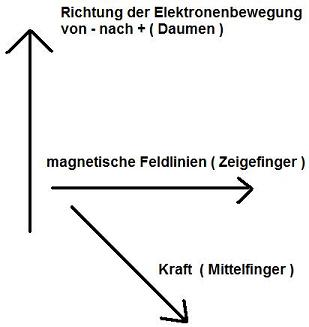Right hand rule:

The thumb shows the direction of the electron flow (from + to -) and represents the cause. The index finger then shows the direction of the magnetic field (from the north pole to the south pole). And the middle finger then points in the direction of the force (so it is the effect).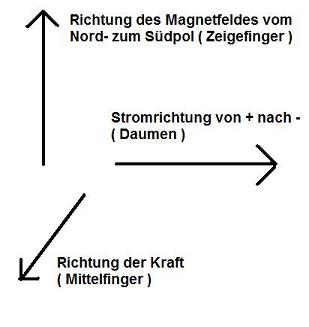Left:

### Who's Online

We have 1721 guests online